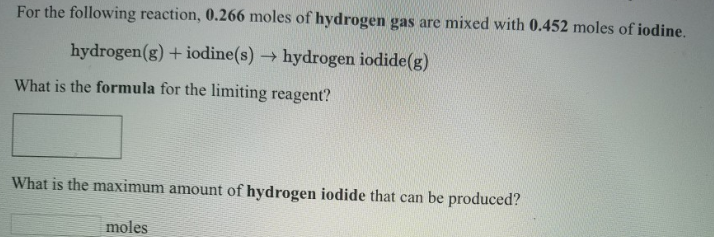# Problem: For the following reaction, 0.266 moles of hydrogen gas are mixed with 0.452 moles of iodine. hydrogen (g) + iodine (s) → hydrogen iodide (g) What is the formula for the limiting reagent? What is the maximum amount of hydrogen iodide that can be produced?

###### FREE Expert Solution
94% (483 ratings)###### Problem Details

For the following reaction, 0.266 moles of hydrogen gas are mixed with 0.452 moles of iodine.

hydrogen (g) + iodine (s) → hydrogen iodide (g)

What is the formula for the limiting reagent?

What is the maximum amount of hydrogen iodide that can be produced?